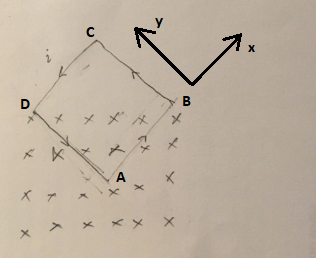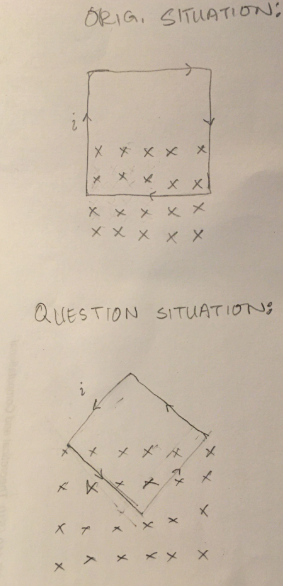# Problem: Consider the wire loop you used in part A. For the lab, you placed the bottom section of the loop in the magnetic field (between two magnets in a box with opening in between for loop to go in) and measured the force upon it when current was flowing through the wire. Imagine instead that both the bottom and one side of the loop were placed in the magnetic field (but not the top or other side). What would be the net direction of the force upon this loop? Explain carefully and refer back to equations if needed. Please specify the NET direction!

###### FREE Expert Solution

Force on a current-carrying conductor:

$\overline{){\mathbf{F}}{\mathbf{=}}{\mathbf{B}}{\mathbf{i}}{\mathbf{L}}{\mathbf{s}}{\mathbf{i}}{\mathbf{n}}{\mathbf{\theta }}}$

The angle between the current and the magnetic field is 90°. Thus:

$\overline{){\mathbf{F}}{\mathbf{=}}{\mathbf{B}}{\mathbf{i}}{\mathbf{L}}}$Right-hand rule for currents in B-fields: Fingers→ field direction, Thumb→ current direction, and palm→ force direction.

FAD is directed in the positive x-axis.

FAB is directed in the positive y-axis.

83% (435 ratings)###### Problem Details

Consider the wire loop you used in part A. For the lab, you placed the bottom section of the loop in the magnetic field (between two magnets in a box with opening in between for loop to go in) and measured the force upon it when current was flowing through the wire. Imagine instead that both the bottom and one side of the loop were placed in the magnetic field (but not the top or other side). What would be the net direction of the force upon this loop? Explain carefully and refer back to equations if needed. Please specify the NET direction!